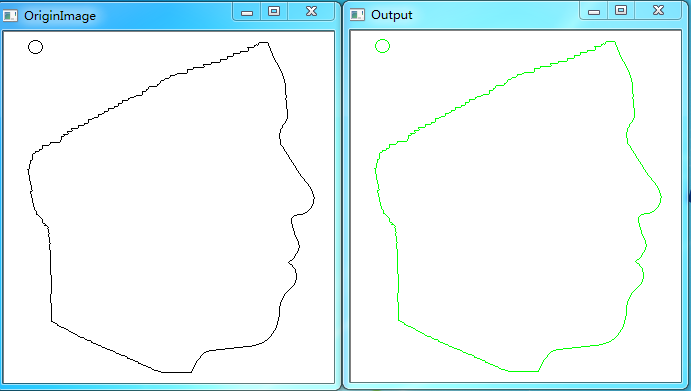# 图像处理 边界跟踪

## matlab图像处理之二值图像内外边界跟踪

2017-03-09 10:40:26 zhangyibo123456789 阅读数 7373
• ###### matlab边缘检测和图像分割

matlab边缘检测 <img src="https://img-bss.csdn.net/201903190616489027.jpg" alt="" /></p>

7课时 101分钟 981人学习 刘昱显
免费试看

# matlab图像处理之二值图像内外边界跟踪

目标内边界的像素全都在目标里面，目标外边界的像素全都不在目标上，是包围着目标的。

首先是内边界跟踪：

1.遍历图像。
2.标记第一个遇见像素块的前景像素（i,j）。
3.对这个像素周围八邻域逆时针搜索，如果搜索到周围有前景像素，那么更新坐标（i,j）为（i',j'），并标记。
4.不断执行第3步直到再次遇见此像素块第一次标记的像素。
5.继续执行第1步。

然后是外边界跟踪：

第二种也不算跟踪方法，只是标记算法而已。就是将图像中前景像素周围的非前景像素标记一下就行了。matlab程序如下：

clear all;
close all;
clc;

img=img>128;
imshow(img);
[m n]=size(img);

imgn=zeros(m,n);        %边界标记图像
ed=[-1 -1;0 -1;1 -1;1 0;1 1;0 1;-1 1;-1 0]; %从左上角像素，逆时针搜索
for i=2:m-1
for j=2:n-1
if img(i,j)==1 && imgn(i,j)==0      %当前是没标记的白色像素
if sum(sum(img(i-1:i+1,j-1:j+1)))~=9    %块内部的白像素不标记
ii=i;         %像素块内部搜寻使用的坐标
jj=j;
imgn(i,j)=2;    %本像素块第一个标记的边界，第一个边界像素为2

while imgn(ii,jj)~=2    %是否沿着像素块搜寻一圈了。
for k=1:8           %逆时针八邻域搜索
tmpi=ii+ed(k,1);        %八邻域临时坐标
tmpj=jj+ed(k,2);
if img(tmpi,tmpj)==1 && imgn(tmpi,tmpj)~=2  %搜索到新边界，并且没有搜索一圈
ii=tmpi;        %更新内部搜寻坐标，继续搜索
jj=tmpj;
imgn(ii,jj)=1;  %边界标记图像该像素标记，普通边界为1
break;
end
end
end

end
end
end
end

figure;
imgn=imgn>=1;
imshow(imgn,[]);

%不过要是真取二值图像内边界，通常是原图减去其腐蚀图就行了
se = strel('square',3);
imgn=img-imerode(img,se);
figure;
imshow(imgn)


外边界：
clear all;
close all;
clc;

img=img>128;
imshow(img);
[m n]=size(img);

imgn=zeros(m,n);        %边界标记图像
ed=[-1 -1;0 -1;1 -1;1 0;1 1;0 1;-1 1;-1 0]; %从左上角像素判断
for i=2:m-1
for j=2:n-1
if img(i,j)==1      %如果当前像素是前景像素

for k=1:8
ii=i+ed(k,1);
jj=j+ed(k,2);
if img(ii,jj)==0    %当前像素周围如果是背景，边界标记图像相应像素标记
imgn(ii,jj)=1;
end
end

end
end
end

figure;
imshow(imgn,[]);

%不过要是真取二值图像外边界，通常是原图膨胀图减去原图就行了
se = strel('square',3);
imgn=imdilate(img,se)-img;
figure;
imshow(imgn)

## 图像处理基础知识系列之一：边界跟踪之内边界跟踪算法解释

2017-05-26 19:10:29 coming_is_winter 阅读数 12409
• ###### matlab边缘检测和图像分割

matlab边缘检测 <img src="https://img-bss.csdn.net/201903190616489027.jpg" alt="" /></p>

7课时 101分钟 981人学习 刘昱显
免费试看

# 边界跟踪：内边界跟踪算法解释

边界跟踪是基于边缘的分割常用方法之一，用于区域已分出（二值或已标注），但边界未知的情况。分为内边界与外边界。内边界为区域的一个子集，外边界不是区域的一个子集。

内边界跟踪算法具体的实施方法比较明确，但在学习的时候感觉稍微有点抽象，结合自己的理解拓展一下。
具体算法：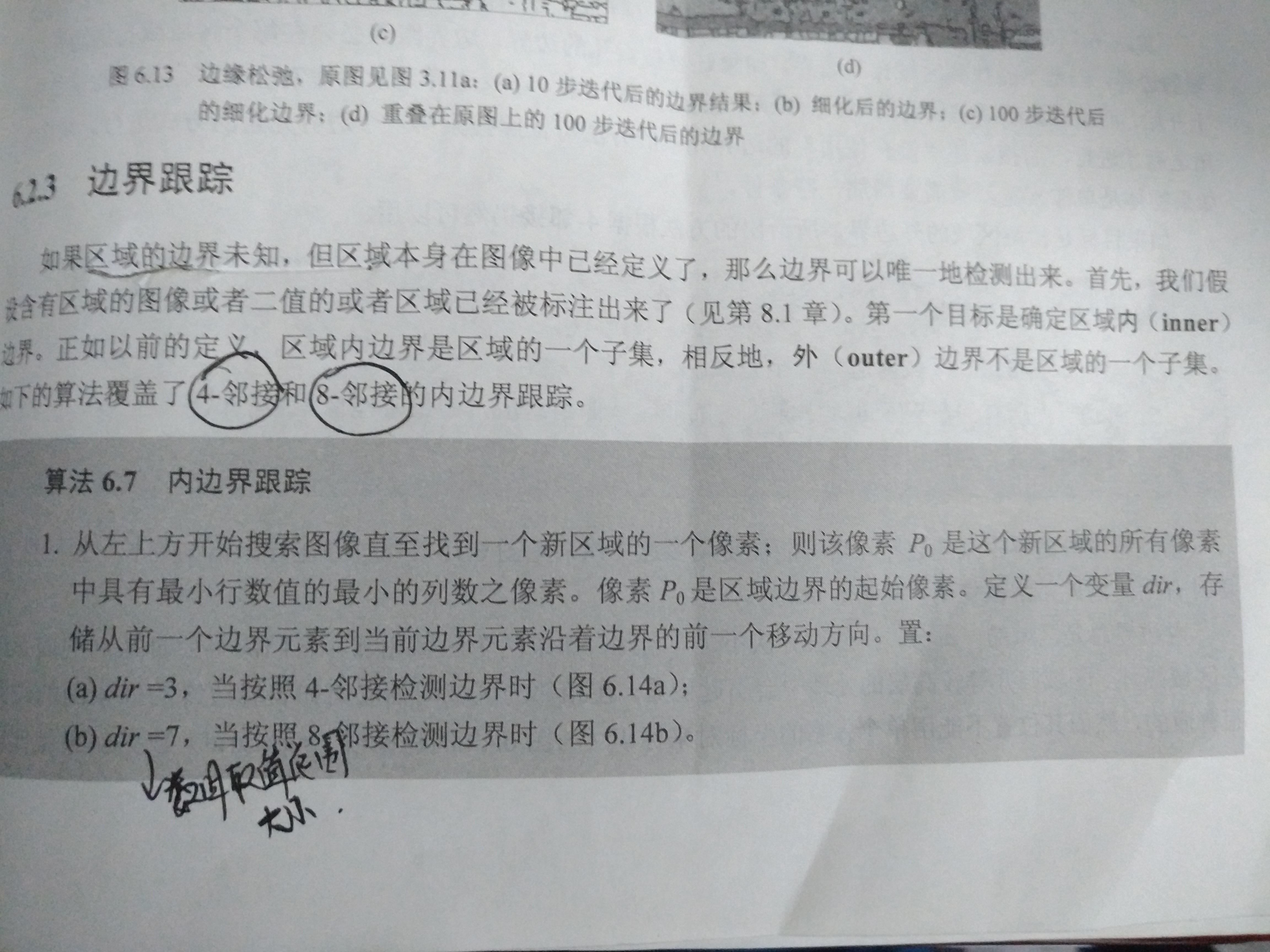图1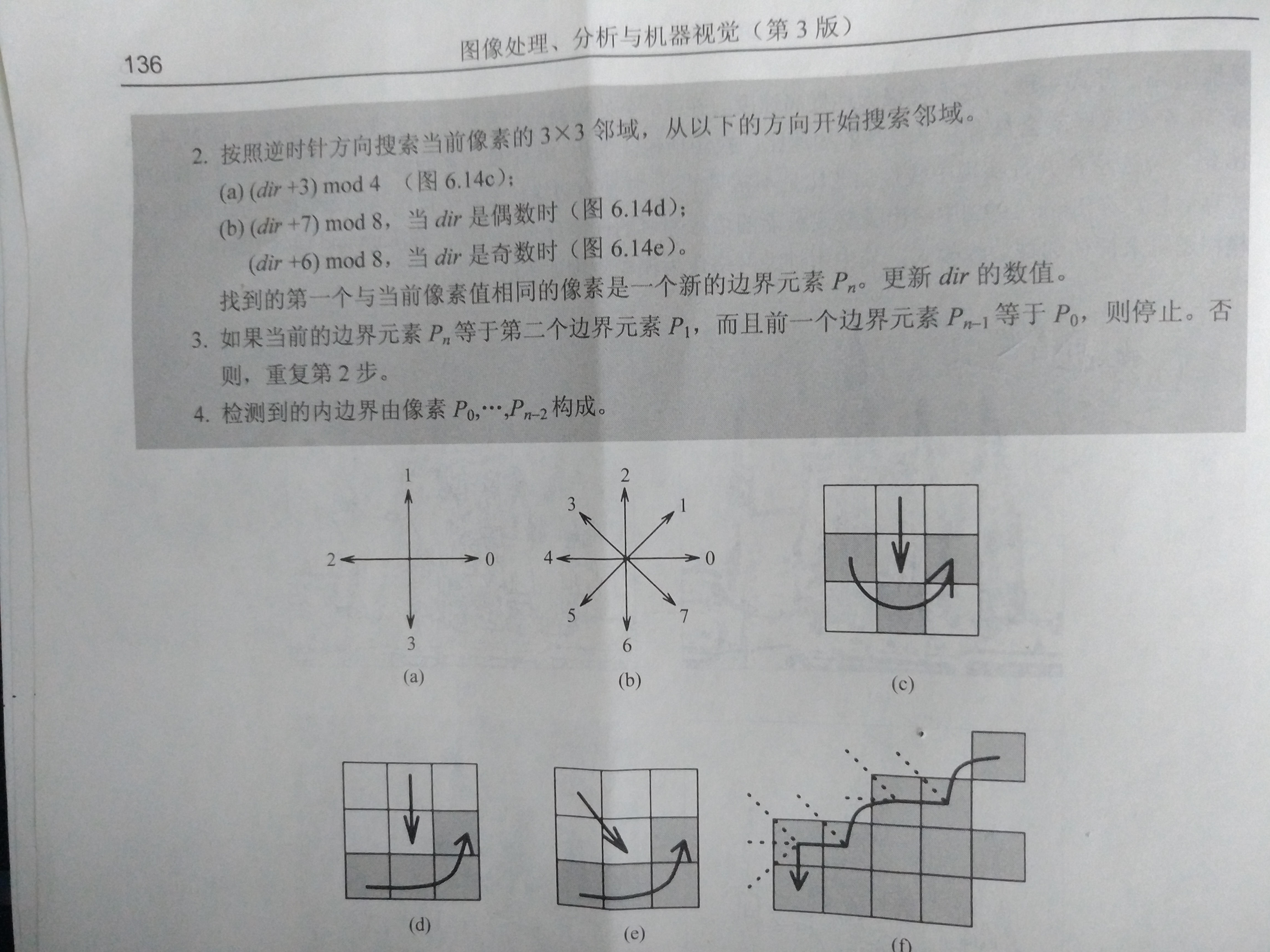图2

具体有四步，以上图片中包含四邻域与八邻域，下面主要以以八邻域为例，四邻域原理相似。

第一步中图像处理是以整个图片的最左上角为坐标原点（0,0），所以最靠近左上角的P0为最小行数与最小列数之像素。定义的dir为边界移动的方向，具体为八邻域中的八个方向（0,1,2,3,4,5,6,7）中的一个（如图2中的（b）图），此时默认初始值为7（右下方向）。

第二步中先判断dir的奇偶性（默认值为7），然后对dir进行计算更改（就是根据上下文调整邻域扫描的初始方向））进行下一轮边界扫描。具体操作看图，画的不是很好，有点乱。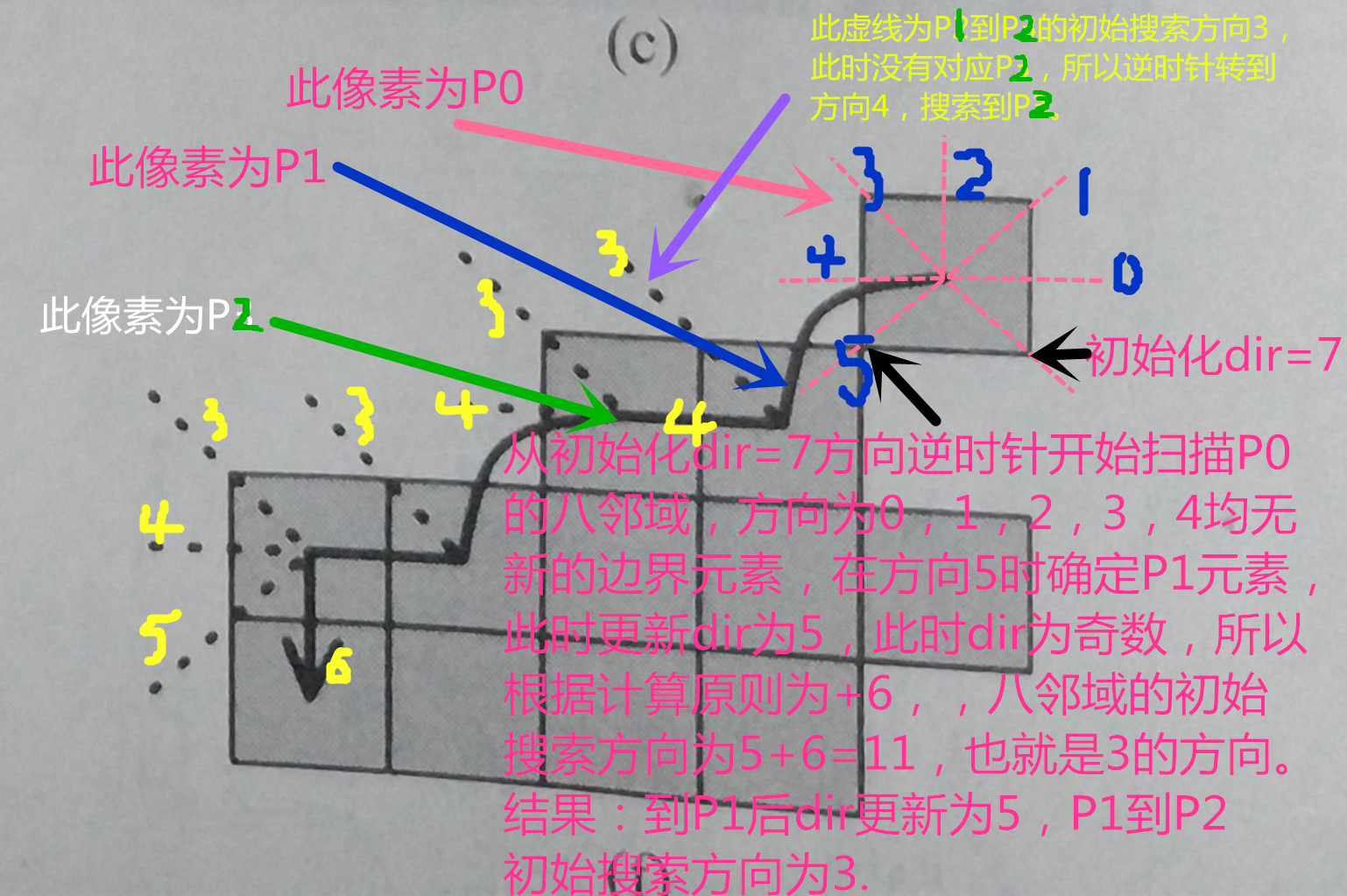这里的奇偶判断及为何是+6，+7具体原理尚未能有效想明白，有待进一步学习。

第三步比较好理解边界是一个闭合空间，搜索到首尾相接时即结束。

第四步及将计算了两遍的Pn-1与Pn删除，最后边界为P0到Pn-2。

水平有限，理解可能有误，加之文笔不好，凑合看哈。

PS:后边发现有一个不确定的地方，搜索P0的时候dir是默认的7还是先判断dir的奇偶性再按第二步的步骤进行呢？这里先按初始7计算。

参考文献：

《图像处理、分析与机器视觉（第三版）》     作者 ：Milan Sonka、艾海舟。

## 图像处理之边界跟踪

2017-11-10 21:00:36 hustllx 阅读数 1254
• ###### matlab边缘检测和图像分割

matlab边缘检测 <img src="https://img-bss.csdn.net/201903190616489027.jpg" alt="" /></p>

7课时 101分钟 981人学习 刘昱显
免费试看

matlab函数：B=bwboundaries(img)；

close all;clear all;
img = rgb2gray(img);
img = im2bw(img);
img=im2bw(img);
B=bwboundaries(img);%库函数结果

[m,n]=size(img);
k=1;
flag=0;
for i=1:m %找到第一个不为0元素位置
for j=1:n
if img(i,j)>0
stack(k,1)=i;
stack(k,2)=j;
starti=i;   %标记第一个点
startj=j;
dir=7;
k=k+1;
flag=1; %表示已经找到了第一个元素
break;
end
end
if flag==1
break;
end
end

while 1
if mod(dir,2)==0
dir=mod(dir+7,8); %偶数处理方式
else
dir=mod(dir+6,8); %奇数的处理方式
end
i=stack(k-1,1);
j=stack(k-1,2);
while 1
flag=1;
switch dir
case 0
if j+1<n+1&&img(i,j+1)>0%右方
stack(k,1)=i;
stack(k,2)=j+1;
k=k+1;
flag=0;%找到下一个元素位置
else
dir=mod(dir+1,8);
end
case 1
if i-1>0&&j+1<n+1&&img(i-1,j+1)>0%右上方
stack(k,1)=i-1;stack(k,2)=j+1;
k=k+1;
flag=0;%找到下一个元素位置
else
dir=mod(dir+1,8);
end
case 2
if i-1>0&&img(i-1,j)>0%上方
stack(k,1)=i-1;stack(k,2)=j;
k=k+1;
flag=0;%找到下一个元素位置
else
dir=mod(dir+1,8);
end
case 3
if i-1>0&&j-1>0&&img(i-1,j-1)>0%左上方
stack(k,1)=i-1;stack(k,2)=j-1;
k=k+1;
flag=0;%找到下一个元素位置
else
dir=mod(dir+1,8);
end
case 4
if j-1>0&&img(i,j-1)>0%左方
stack(k,1)=i;stack(k,2)=j-1;
k=k+1;
flag=0;%找到下一个元素位置
else
dir=mod(dir+1,8);
end
case 5
if i+1<m+1&&j-1>0&&img(i+1,j-1)>0%左下方
stack(k,1)=i+1;stack(k,2)=j-1;
k=k+1;
flag=0;%找到下一个元素位置
else
dir=mod(dir+1,8);
end
case 6
if i+1<m+1&&img(i+1,j)>0%下方
stack(k,1)=i+1;stack(k,2)=j;
k=k+1;
flag=0;%找到下一个元素位置
else
dir=mod(dir+1,8);
end
case 7
if i+1<m+1&&j+1<n+1&&img(i+1,j+1)>0%右下方
stack(k,1)=i+1;stack(k,2)=j+1;
k=k+1;
flag=0;%找到下一个元素位置
else
dir=mod(dir+1,8);
end
otherwise
end
if flag==0%找到下一个点退出当前循环
break;
end
end
if  k-1>2&&stack(k-2,1)==starti&&stack(k-2,2)==startj&&stack(k-1,1)==stack(2,1)&&stack(k-1,2)==stack(2,2)%退出循环条件
break;
end
end
stack(k-1,:)=[];

figure;
subplot(1,2,1);
imshow(img);
subplot(1,2,2);
imshow(img);
hold on;
for i=1:length(stack)
hold on; plot(stack(:,2),stack(:,1),'r');
end

## 图像预处理——边界跟踪、图像分割

2017-05-14 22:19:10 PinkRiverside 阅读数 1265
• ###### matlab边缘检测和图像分割

matlab边缘检测 <img src="https://img-bss.csdn.net/201903190616489027.jpg" alt="" /></p>

7课时 101分钟 981人学习 刘昱显
免费试看P(x,y)$P(x,y)$ 为物体的一个边界点，则P(x,y)$P(x,y)$ 的下一个边界点必存在其八邻域内，因此可以根据八邻域信息进行外边界跟踪。在找到下一个边界点后，依次边界点为当前边界点继续搜索。这一搜索过程不断重复下去，直到搜索至起点。## 形态学图像处理之边界提取与跟踪

2019-08-11 22:07:58 webzhuce 阅读数 1231
• ###### matlab边缘检测和图像分割

matlab边缘检测 <img src="https://img-bss.csdn.net/201903190616489027.jpg" alt="" /></p>

7课时 101分钟 981人学习 刘昱显
免费试看

# 边界提取

要在二值图像中提取物体的边界，容易想到的一个方法是将所有物体内部的点删除（置为背景色）。具体地说，可以逐行扫描图像，如果发现一个黑点的8个邻域都是黑点，则该点为内部点，在目标图 像中将它删除。实际上这相当于采用一个3*3的结构元素对原图进行腐蚀，使得只有那些8个邻域都有黑点的内部点被保留，再用原图像减去腐蚀后的图像，恰好删除了这些内部点，留下了边界像素。这过程如下图所示。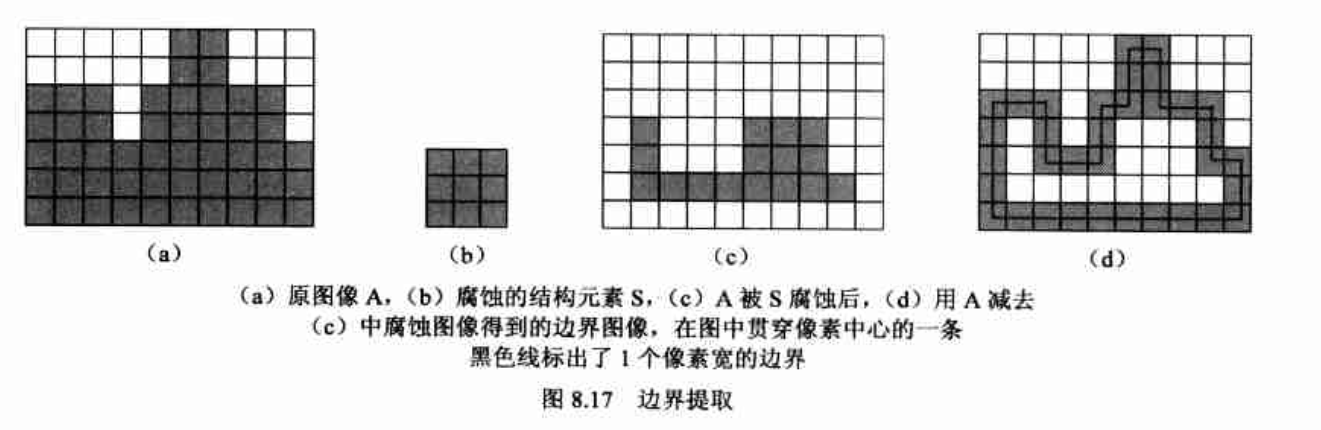## 示例演示

利用OpenCV实现上面边界提取的功能。

#include<opencv2/opencv.hpp>
using namespace cv;

int main(int argc, char *argv[])
{
Mat element = getStructuringElement(MORPH_RECT, Size(3, 3));
imshow("OriginImage", originimage);
Mat erodeimage;
cv::erode(originimage, erodeimage, element);
imshow("ErodeImage", erodeimage);

Mat output = originimage -  erodeimage;
imshow("Output", output);

waitKey(0);
return 0;

}


## 运行结果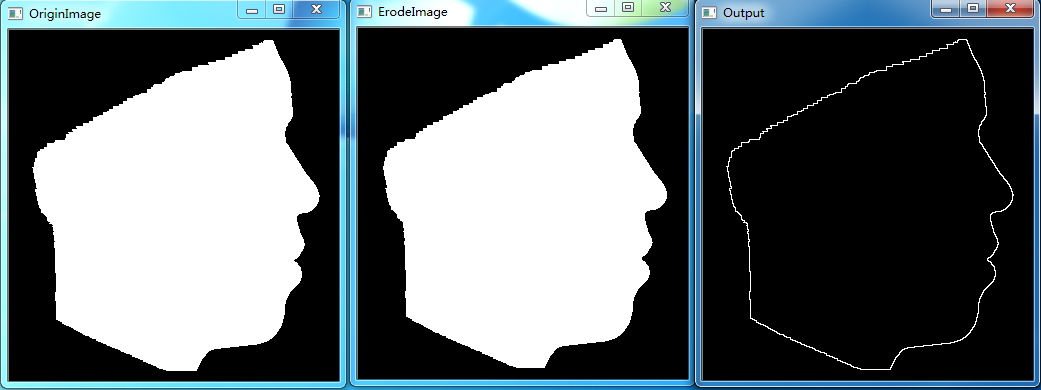# 边界跟踪

为了依次记录边界上的每个像素，边界跟踪首先按照某种扫描规则找到目标物体边界上的一个像素，然后就以该像素为起始点，根据某种顺序（如顺时针或逆时针）依次找出物体边界上的其余像素，直到又回到起始点，完成整条边界的跟踪。

## 示例演示

在一幅图像中，实现跟踪多个边界的功能。对于带孔洞的物体也可以跟踪至其孔洞的轮廓。

#include<opencv2/opencv.hpp>
using namespace cv;

//only process binary image
//black is boundary
std::vector<std::vector<cv::Point>> TraceBoundary(Mat &image)
{
std::vector<std::vector<Point>> boundaryset;
Point start, current, next; // start point and current point
//search dirction array
int direction ={{-1, 1}, //left-down
{0, 1}, // down
{1, 1}, //right-down
{1, 0}, //right
{1, -1}, //right-up
{0, -1}, //up
{-1, -1}, //left-up
{-1, 0}  // left
};
int begindirection = 0, currentdirection = 0;
bool atstart = false, findboundary = false;

for(int i = 0; i < image.rows; i++)
{
for(int j = 0; j < image.cols; j++)
{
if(image.at<uchar>(i, j) == 0) //find start point
{
start.x = j;
start.y = i;
current = start;
atstart = true;
findboundary =  true;
std::vector<Point> points;
points.push_back(current);
std::cout << "Start: " << j << " " << i << std::endl;
while((current.x != start.x) || (current.y != start.y) || atstart)
{
atstart = false;

//search next point
next.x = current.x + direction[currentdirection];
next.y = current.y + direction[currentdirection];
int searchtimes = 1;
while(next.x < 0 || next.x >= image.cols || next.y < 0 || next.y >= image.rows || image.at<uchar>(next) == 255)
{
currentdirection++; //rotate 45 degrees counterclockwise
currentdirection %= 8;
next.x = current.x + direction[currentdirection];
next.y = current.y + direction[currentdirection];
//there are no boundary points in 8 domains, which means they are isolated points
if(++searchtimes >= 8)
break;
}
if(image.at<uchar>(next) == 0) // find next point
{
std::cout << "Next: " << next.x << " " << next.y << std::endl;
points.push_back(next);
current = next;
currentdirection -= 2;
if(currentdirection < 0)
currentdirection += 8;

}
else // not find next point
{
findboundary = false;
break;
}
}
if(findboundary)
{
boundaryset.push_back(points);
for(auto &p : points)
{
image.at<uchar>(p) = 255;
}
}
} // find boundary one time
} // for j
} // for i
return boundaryset;
}

int main(int argc, char *argv[])
{
imshow("OriginImage", originimage);

Mat image;
cvtColor(originimage, image, CV_BGR2GRAY);
std::vector<std::vector<Point>> boundaryset = TraceBoundary(image);

//show result
Mat result;
originimage.copyTo(result);
for(auto &points : boundaryset)
{
for(auto &p : points)
{
result.at<Vec3b>(p)= 0;
result.at<Vec3b>(p)= 0;
result.at<Vec3b>(p)= 255;
}
}
imshow("Output", result);

waitKey(0);
return 0;

}


## 运行结果